PCA VI

LabVIEW 2018 Analytics and Machine Learning Toolkit Help

Edition Date: July 2018

Part Number: 377059B-01

»View Product InfoDownload Help (Windows Only)

Owning Palette: Feature Manipulation VIs

Requires: Analytics and Machine Learning Toolkit

Trains a principal component analysis (PCA) model. You can use the PCA model to reduce the dimension of training data.

You can perform batch training with this VI when you have a large training data set.

Examples

Use the pull-down menu to select an instance of this VI.

 Select an instance One ShotBatch

One Shot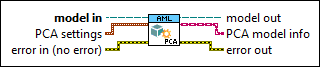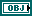model in specifies the information about the entire workflow of the model.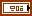PCA settings specifies the method and value for this VI to calculate the number of principal components.criteria specifies the method for this VI to calculate the number of principal components.

 0 number of components (default)—Directly specifies the number of principal components. 1 ratio of variance—Specifies the ratio of variance for this VI to calculate the number of principal components.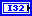number of components specifies the number of principal components. This input is valid only if criteria is number of components.ratio of variance specifies the ratio of the variance in principal components to the total variance. This VI automatically calculates the number of components. The default is 0.95. This input is valid only if criteria is ratio of variance.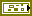error in describes error conditions that occur before this node runs. This input provides standard error in functionality.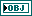model out returns the information about the entire workflow of the model. Wire model out to the reference input of a standard Property Node to get an AML Analytics Property Node.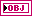PCA model info returns the information about the PCA model. Wire PCA model info to the reference input of a standard Property Node to get an AML PCA Property Node.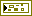error out contains error information. This output provides standard error out functionality.

Batch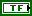reset specifies whether to reset the trained model programmatically at run time. The default is FALSE.model in specifies the information about the entire workflow of the model.number of components specifies the number of principal components. The default is 2. number of components must be reasonable to avoid too much memory usage for a single batch. For the first batch data, number of components must be less than or equal to the number of rows in the training data. Starting from the second batch data, number of components can be 1 or any number that fits in the computation capacity.error in describes error conditions that occur before this node runs. This input provides standard error in functionality.model out returns the information about the entire workflow of the model. Wire model out to the reference input of a standard Property Node to get an AML Analytics Property Node.PCA model info returns the information about the PCA model. Wire PCA model info to the reference input of a standard Property Node to get an AML PCA Property Node.error out contains error information. This output provides standard error out functionality.

Examples

Refer to the following VIs for examples of using the PCA VI:

• Feature Manipulation (Training) VI: labview\examples\AML\Feature Manipulation
• Feature Manipulation (Training) (Batch) VI: labview\examples\AML\Feature Manipulation
• Clustering (Set Parameters, Training) VI: labview\examples\AML\Clustering
• Clustering (Search Parameters, Training) VI: labview\examples\AML\Clustering
• Clustering (Deployment) VI: labview\examples\AML\Clustering